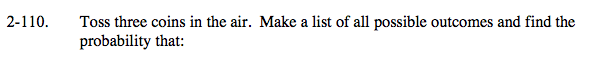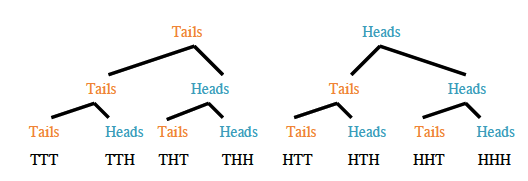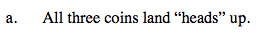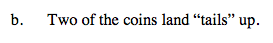### Home > CAAC > Chapter 14 > Lesson 14.2.1.8 > Problem2-110

2-110.
1. Toss three coins in the air. Make a list of all possible outcomes and find the probability that: Homework Help ✎

1. All three coins land “heads” up.

2. Two of the coins land “tails” up.$\frac{1}{8}$$\frac{3}{8}\text{ or }\frac{1}{2} \text{ assuming TTT counts.}$